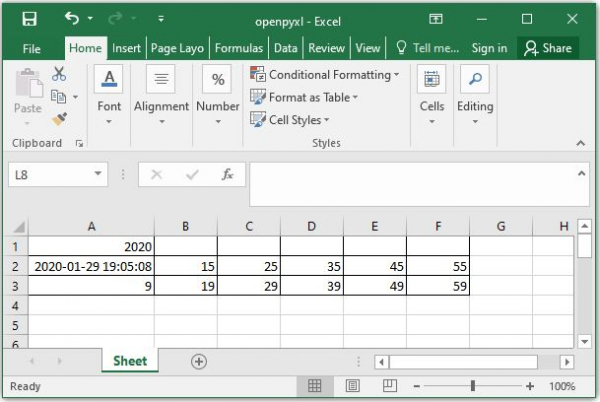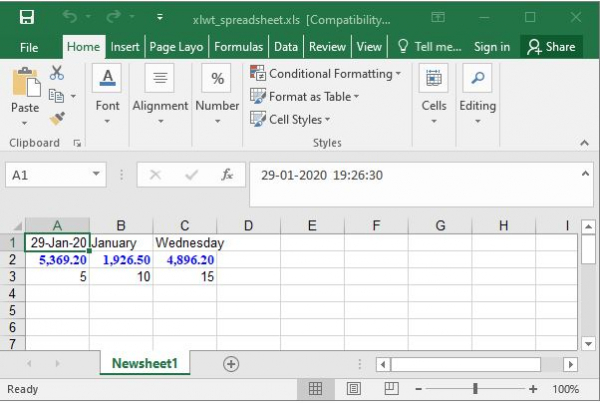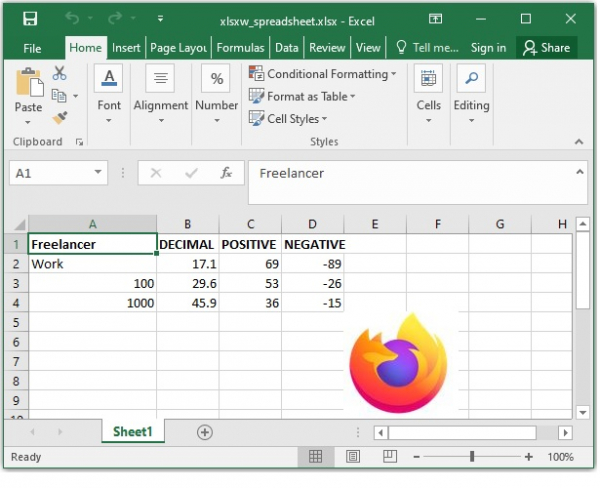Excel is the most famous spreadsheet and almost every computer user is comfortable with the idea of managing the data through spreadsheets. Eventually some python program has to interact with excel. Many python libraries are available to create, read and write into excel files. We will see the examples of few such important libraries below.

## Using openpyxl

This library can read/write Excel 2010 xlsx/xlsm/xltx/xltm files. In the below example we create a excel worksheet, assign data to its cells and finally save the file to a desired location. The module has many in-built methods which can be used for this. We see those methods used below.

## Example

#openpyxl
from openpyxl import Workbook

# grab the active worksheet

# Data can be assigned directly to cells
worksheet['A1'] = 50

# Rows can also be appended
worksheet.append([5, 15, 25, 35, 45, 55])
worksheet.append([9, 19, 29, 39, 49, 59])

# Python types will automatically be converted
import datetime
t1 = datetime.datetime.now()
worksheet['A1'] = t1.year
worksheet['A2'] = t1

# Save the file
Spreadsheet.save("E:\openpyxl.xlsx")

## Output

Running the above code gives us the following result −## Using xlwt

This is a library for developers to use to generate spreadsheet files compatible with Microsoft Excel versions 95 to 2003. We can not only write the values into the cells, we can also format the values. The values can be made bold, coloured, italic etc. Also the font size can be controlled. In the below example we format the numbers written and make them blue coloured.

## Example

import xlwt
from datetime import datetime

format1 = xlwt.easyxf('font: name Times New Roman, color-index blue, bold on', num_format_str='#,##0.00')
format2 = xlwt.easyxf(num_format_str='D-MMM-YY')

worksheet.write(0, 0, datetime.now(), format2)
worksheet.write(0, 1, datetime.now().strftime("%B"),format2)
worksheet.write(0, 2, datetime.now().strftime("%A"),format2)
worksheet.write(1, 0, 5369.2, format1)
worksheet.write(1, 1, 1926.5,format1)
worksheet.write(1, 2, 4896.2,format1)
worksheet.write(2, 0, 5)
worksheet.write(2, 1, 10)
worksheet.write(2, 2, xlwt.Formula("A3+B3"))
Spreadsheet.save('E:\xlwt_spreadsheet.xls')

## Output

Running the above code gives us the following result −## Using xlsxwriter

This module can create excel files of version 2007. It has much wider number of features than the above to excel file creating modules. It can write text, numbers, formulas and hyperlinks to multiple worksheets. In the below example, not only we do the formatting of the text in the sheet, but also we add an image to the sheet.

## Example

import xlsxwriter
sheet.set_column('A:A', 18)

# Add a bold format to use to highlight cells.

#simple text.
sheet.write('A1','Freelancer',bold)
sheet.write('A2', 'Work')
sheet.write('A3', 100)
sheet.write('A4', 1000)

sheet.write('B1', 'DECIMAL', bold)
sheet.write('B2', 17.1)
sheet.write('B3', 29.6)
sheet.write('B4', 45.9)

sheet.write('C1', 'POSITIVE',bold)
sheet.write('C2', 69)
sheet.write('C3', 53)
sheet.write('C4', 36)

sheet.write('D1','NEGATIVE',bold)
sheet.write('D2', -89)
sheet.write('D3', -26)
sheet.write('D4', -15)

# Insert an image.
sheet.insert_image('E5', 'E:\firefox.JPG')
Spreadsheet.close()

## Output

Running the above code gives us the following result −